### IMO Shortlist 2015 problem G1

Kvaliteta:
Avg: 0,0
Težina:
Avg: 6,0

Let$ABC$ be an acute triangle with orthocenter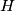$H$. Let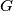$G$ be the point such that the quadrilateral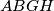$ABGH$ is a parallelogram. Let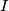$I$ be the point on the line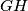$GH$ such that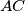$AC$ bisects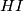$HI$. Suppose that the line$AC$ intersects the circumcircle of the triangle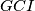$GCI$ at$C$ and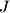$J$. Prove that$IJ = AH$.

(Australia)

Izvor: https://www.imo-official.org/problems/IMO2015SL.pdf